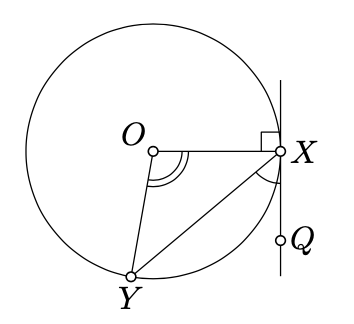Skip to main content
$$\newcommand{\id}{\mathrm{id}}$$ $$\newcommand{\Span}{\mathrm{span}}$$ $$\newcommand{\kernel}{\mathrm{null}\,}$$ $$\newcommand{\range}{\mathrm{range}\,}$$ $$\newcommand{\RealPart}{\mathrm{Re}}$$ $$\newcommand{\ImaginaryPart}{\mathrm{Im}}$$ $$\newcommand{\Argument}{\mathrm{Arg}}$$ $$\newcommand{\norm}{\| #1 \|}$$ $$\newcommand{\inner}{\langle #1, #2 \rangle}$$ $$\newcommand{\Span}{\mathrm{span}}$$

# 9.1: Angle between a tangent line and a chord

$$\newcommand{\vecs}{\overset { \rightharpoonup} {\mathbf{#1}} }$$ $$\newcommand{\vecd}{\overset{-\!-\!\rightharpoonup}{\vphantom{a}\smash {#1}}}$$$$\newcommand{\id}{\mathrm{id}}$$ $$\newcommand{\Span}{\mathrm{span}}$$ $$\newcommand{\kernel}{\mathrm{null}\,}$$ $$\newcommand{\range}{\mathrm{range}\,}$$ $$\newcommand{\RealPart}{\mathrm{Re}}$$ $$\newcommand{\ImaginaryPart}{\mathrm{Im}}$$ $$\newcommand{\Argument}{\mathrm{Arg}}$$ $$\newcommand{\norm}{\| #1 \|}$$ $$\newcommand{\inner}{\langle #1, #2 \rangle}$$ $$\newcommand{\Span}{\mathrm{span}}$$ $$\newcommand{\id}{\mathrm{id}}$$ $$\newcommand{\Span}{\mathrm{span}}$$ $$\newcommand{\kernel}{\mathrm{null}\,}$$ $$\newcommand{\range}{\mathrm{range}\,}$$ $$\newcommand{\RealPart}{\mathrm{Re}}$$ $$\newcommand{\ImaginaryPart}{\mathrm{Im}}$$ $$\newcommand{\Argument}{\mathrm{Arg}}$$ $$\newcommand{\norm}{\| #1 \|}$$ $$\newcommand{\inner}{\langle #1, #2 \rangle}$$ $$\newcommand{\Span}{\mathrm{span}}$$

Theorem $$\PageIndex{1}$$

Let $$\Gamma$$ be a circle with the center $$O$$. Assume the line $$(XQ)$$ is tangent to $$\Gamma$$ at $$X$$ and $$[XY]$$ is a chord of $$\Gamma$$. Then

$2 \cdot \measuredangle QXY \equiv \measuredangle XOY.$

Equivalently,

$$\measuredangle QXY \equiv \dfrac{1}{2} \cdot \measuredangle XOY$$     or     $$\measuredangle QXY \equiv \dfrac{1}{2} \cdot \measuredangle XOY + \pi.$$

Proof

Note that $$\triangle XOY$$ is isosceles. Therefore, $$\measuredangle YXO = \measuredangle OYX$$.

Applying Theorem 7.4.1 to $$\triangle XOY$$, we get$$\begin{array} {rcl} {\pi} & \equiv & {\measuredangle YXO + \measuredangle OYX + \measuredangle XOY \equiv} \\ {} & \equiv & {2 \cdot \measuredangle YXO + \measuredangle XOY.} \end{array}$$

By Lemma 5.6.2, $$(OX) \perp (XQ)$$, Therefore,

$$\measuredangle QXY + \measuredangle YXO \equiv \pm \dfrac{\pi}{2}.$$

Therefore,

$$2 \cdot \measuredangle QXY \equiv \pi - 2 \cdot YXO \equiv \measuredangle XOY.$$